# Department of Mechanical Engineering ME 322 Mechanical Engineering

• Slides: 18Department of Mechanical Engineering ME 322 – Mechanical Engineering Thermodynamics Lecture 20 Entropy Balance EquationThe Second Law of Thermodynamics Entropy is a balanced quantity. Therefore, Entropy transported into a system - Entropy transported out of a system + Entropy produced within a system = Entropy gained within a system Total Entropy Form Entropy Rate Form Something to think about. . . We have already seen that entropy production occurs due to irreversibility. But, how is entropy transported across a system boundary? 2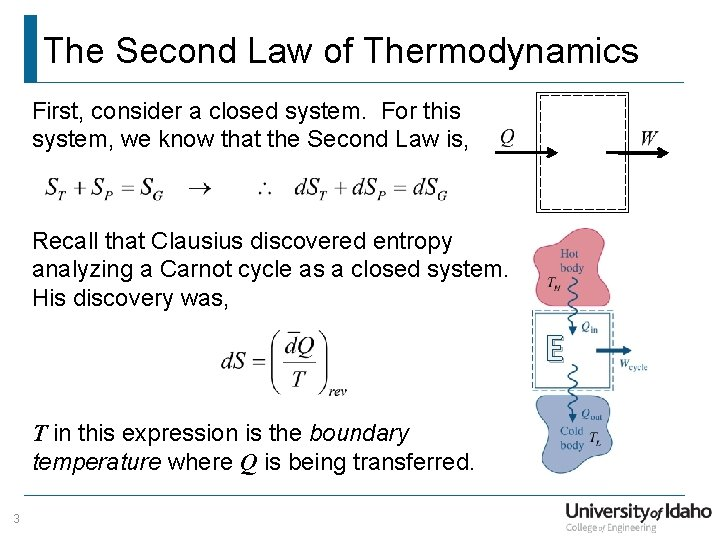The Second Law of Thermodynamics First, consider a closed system. For this system, we know that the Second Law is, Recall that Clausius discovered entropy analyzing a Carnot cycle as a closed system. His discovery was, E T in this expression is the boundary temperature where Q is being transferred. 3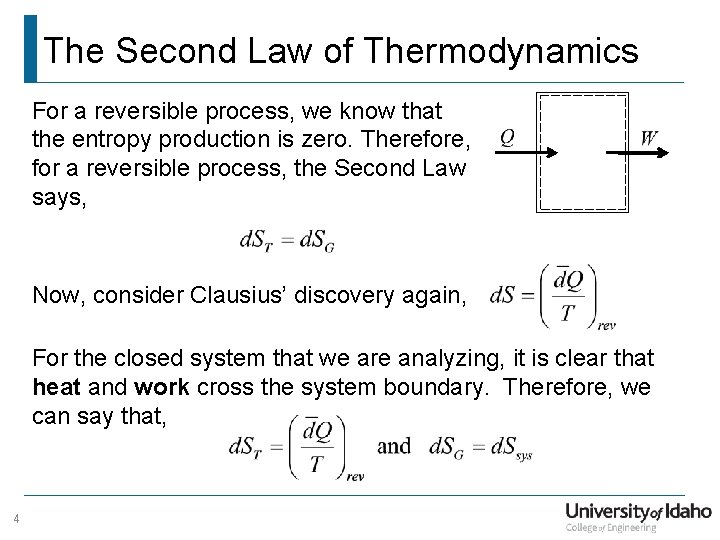The Second Law of Thermodynamics For a reversible process, we know that the entropy production is zero. Therefore, for a reversible process, the Second Law says, Now, consider Clausius’ discovery again, For the closed system that we are analyzing, it is clear that heat and work cross the system boundary. Therefore, we can say that, 4The Second Law of Thermodynamics We have just discovered that the entropy is transported into the system by heat, but NOT by work! For any closed system process, we can write, This expression can now be integrated between any two states, 5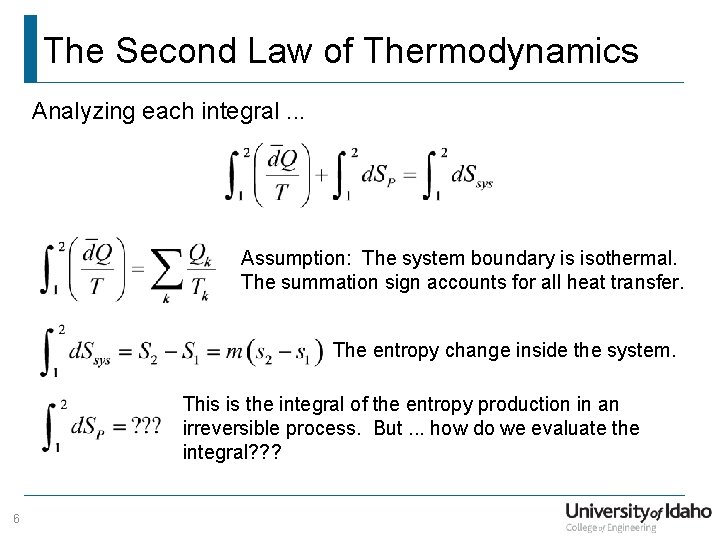The Second Law of Thermodynamics Analyzing each integral. . . Assumption: The system boundary is isothermal. The summation sign accounts for all heat transfer. The entropy change inside the system. This is the integral of the entropy production in an irreversible process. But. . . how do we evaluate the integral? ? ? 6The Second Law of Thermodynamics Entropy production is caused by irreversibilities. Consider two piston-cylinder assemblies. They are identical in every way except that one of them operates reversibly and the other is irreversible. Questions. . . reversible 7 irreversible 1. Which system has zero entropy production? 2. Which system delivers more work? 3. Is work a property? 4. Is entropy production a property? 5. Didn’t the Kelvin-Planck Statement say what was on the left was impossible?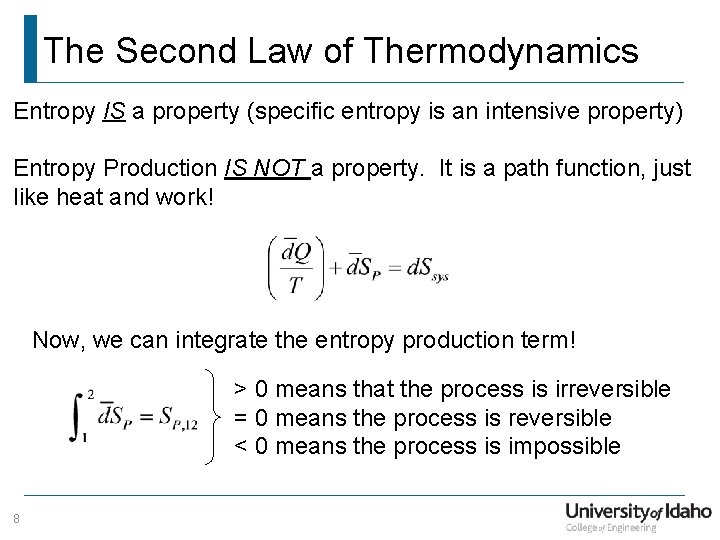The Second Law of Thermodynamics Entropy IS a property (specific entropy is an intensive property) Entropy Production IS NOT a property. It is a path function, just like heat and work! Now, we can integrate the entropy production term! > 0 means that the process is irreversible = 0 means the process is reversible < 0 means the process is impossible 8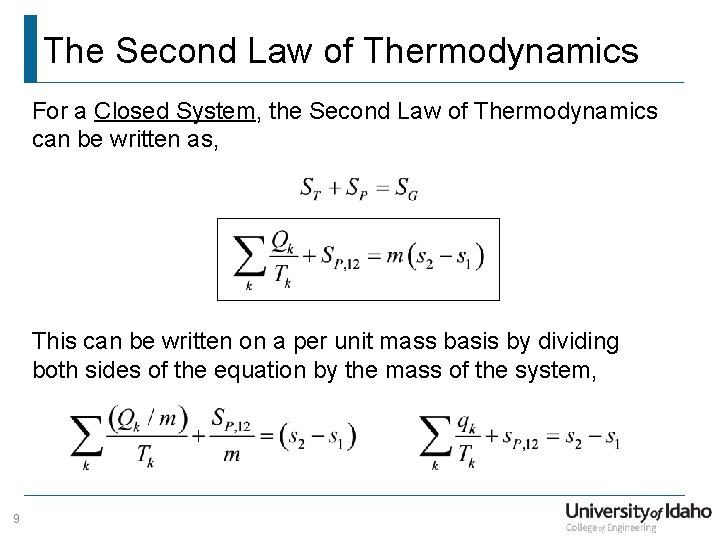The Second Law of Thermodynamics For a Closed System, the Second Law of Thermodynamics can be written as, This can be written on a per unit mass basis by dividing both sides of the equation by the mass of the system, 9The Second Law of Thermodynamics Incorporating the net entropy transport into the system due to the mass flows gives the complete form of the Second Law of Thermodynamics! Notice that if the system is closed, 10The Laws of the Universe Conservation of Mass – The Continuity Equation Conservation of Energy – The First Law of Thermodynamics The Entropy Balance – The Second Law of Thermodynamics 11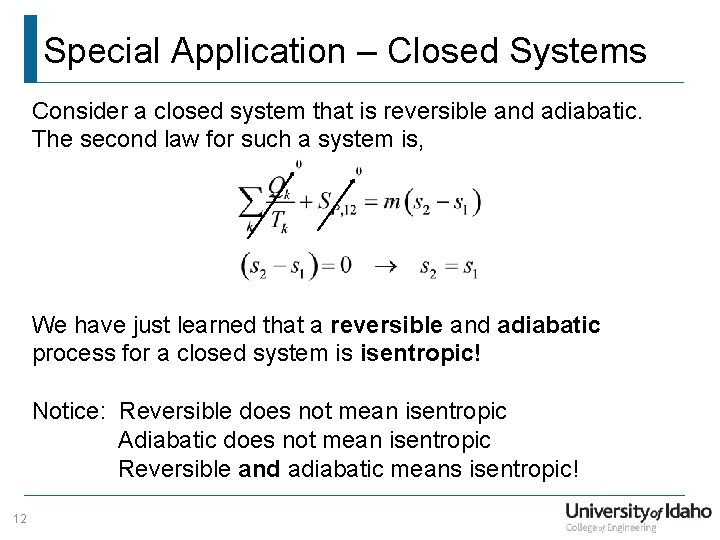Special Application – Closed Systems Consider a closed system that is reversible and adiabatic. The second law for such a system is, We have just learned that a reversible and adiabatic process for a closed system is isentropic! Notice: Reversible does not mean isentropic Adiabatic does not mean isentropic Reversible and adiabatic means isentropic! 12Special Application – Open Systems Consider an open system that is operating in steady state and is reversible and adiabatic with one flow in and one flow out. The second law for such a system is, We have just showed that a reversible and adiabatic are the conditions for isentropic for either open or closed systems. 13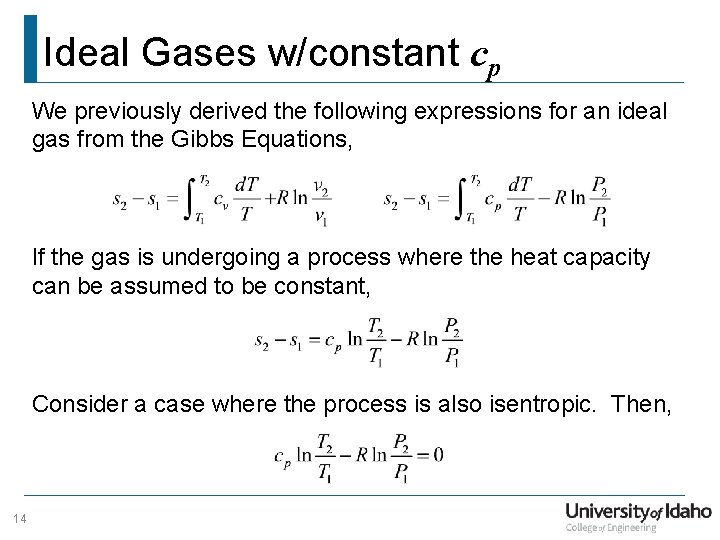Ideal Gases w/constant cp We previously derived the following expressions for an ideal gas from the Gibbs Equations, If the gas is undergoing a process where the heat capacity can be assumed to be constant, Consider a case where the process is also isentropic. Then, 14Ideal Gases w/constant cp Algebra time. . . Rearrange the exponent, 15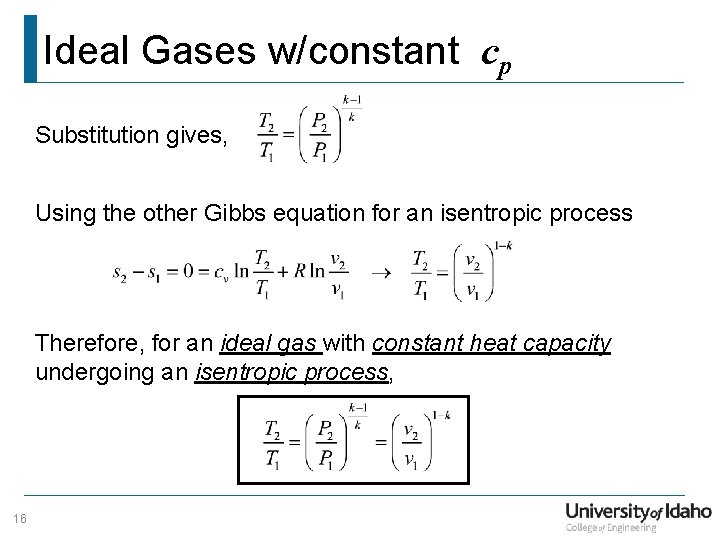Ideal Gases w/constant cp Substitution gives, Using the other Gibbs equation for an isentropic process Therefore, for an ideal gas with constant heat capacity undergoing an isentropic process, 16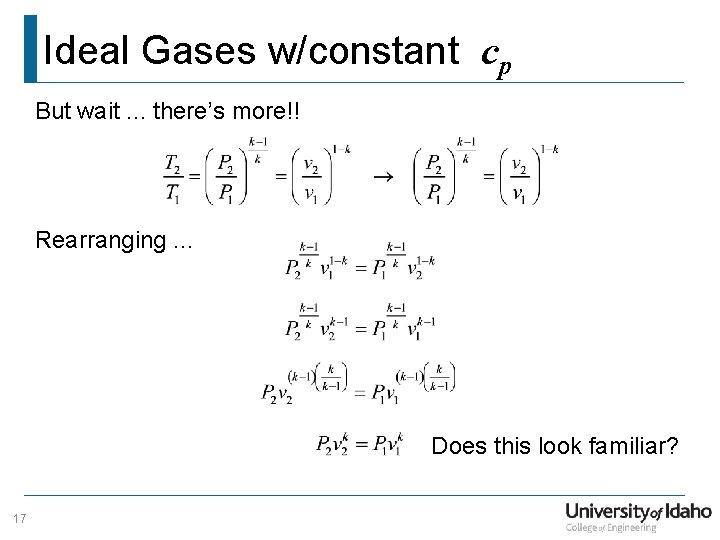Ideal Gases w/constant cp But wait. . . there’s more!! Rearranging. . . Does this look familiar? 17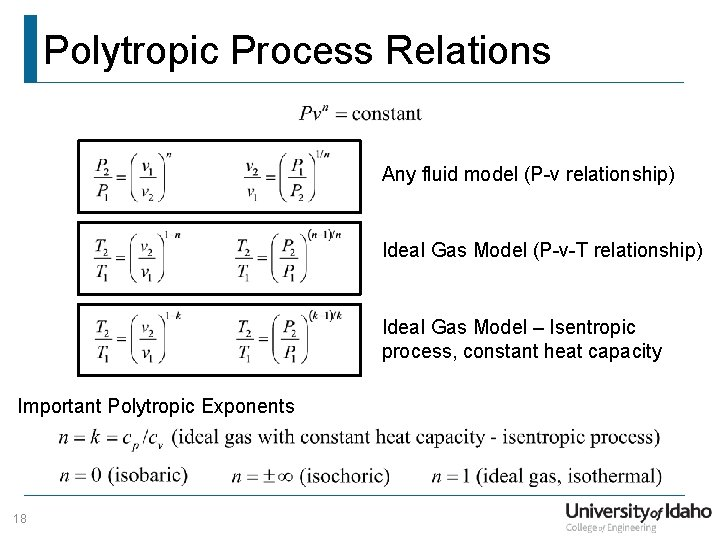Polytropic Process Relations Any fluid model (P-v relationship) Ideal Gas Model (P-v-T relationship) Ideal Gas Model – Isentropic process, constant heat capacity Important Polytropic Exponents 18